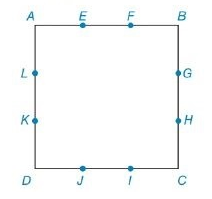Chapter 8.2, Problem 42EElementary Geometry For College St...

7th Edition
Alexander + 2 others
ISBN: 9781337614085

Solutions

Chapter
SectionElementary Geometry For College St...

7th Edition
Alexander + 2 others
ISBN: 9781337614085
Textbook Problem

For Exercises 41 and 42, the sides of square ABCD are trisected at the indicated points.Find the ratio: a) P E H I L P A B C D b) A E H I L A A B C DExercises 41 and 42

To determine

(a)

To Find:

Ratio of the perimeter of the rectangle EHIL to the square ABCD.

Explanation

Formula Used:

1. Pythagorean theorem for the right angle triangle ABC for the hypotenuse AC,

AC2=AB2+BC2.

2. The perimeter of a polygon is the sum of the lengths of all sides of the polygon. P=4s, where s is side length of the square and P=2b+h, where b is the base and h is the height of the rectangle.

Calculation:

It is given that the sides of square ABCD are trisected as shown in the below diagram.

Let the side length of the square be 3x units. Then the each part will measure x units.

Here EHIL is a rectangle, because the inner square EHIL intersects the outer square in such a way that opposite sides will be in same measure with all the corner angle is a right angle.

As EGIK is a square, each side of ABCD can also be split into two parts. That is, x and 2x respectively. Therefore, AE=BG=IC=DK=x and EB=GC=ID=KA=2x.

Consider the triangle LAE,

Applying Pythagorean Theorem,

LE2=LA2+AE2

LE2=(x)2+

To determine

(b)

To find:

Ratio of the area of the rectangle EHIL to the square ABCD.

Still sussing out bartleby?

Check out a sample textbook solution.

See a sample solution

The Solution to Your Study Problems

Bartleby provides explanations to thousands of textbook problems written by our experts, many with advanced degrees!

Get Started